## CURRICULUM LESSONS

Click the buttons here to skip to the right section, or scroll down to view all my lessons.

NumberPlace Value

#### Place value of positive integersMultiples, Factors and Primes

#### Factors and primes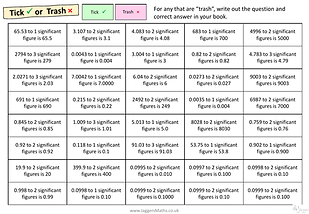Rounding, Estimation and Bounds

#### Rounding to significant figuresFractions

#### Simplifying FractionsFractionsPercentages

#### Percentage increase/decrease with a calculatorStandard form

#### Writing and ordering standard form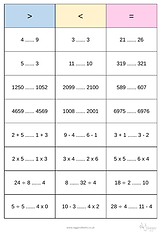Place Value

#### Comparing and ordering positive integersMultiples, Factors and Primes

#### Highest common factorNegative numbers

#### Adding and subtracting negative numbersFractions

#### Simplifying Fractions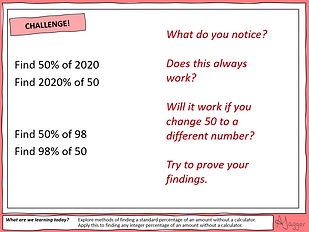Percentages

#### Percentage increase/decrease without a calculatorEquivalent FDP

#### Equivalent FDP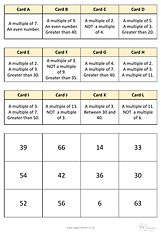Multiples, Factors and Primes

#### Lowest common multipleMultiples, Factors and Primes

#### Product of prime factorsFractions

#### Equivalent Fractions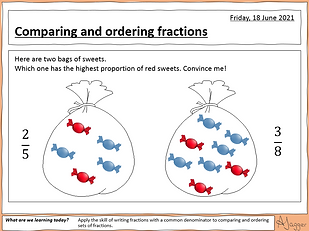Fractions

#### Comparing and ordering fractionsPercentages

#### Percentage of an amount without a calculatorEquivalent FDP

#### Equivalent FDP (including recurring)

Ratio and ProportionProportionCompound Measures

#### Speed, Distance and Time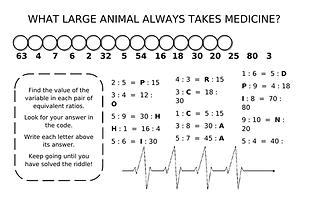Ratio

#### Equivalent RatiosCompound Measures

#### Pressure, Force and AreaRatio

#### Simplifying RatiosCompound Measures

#### Density, Mass and Volume

AlgebraGeometry and MeasuresUnits of measure

#### Convert between metric unitsVolume and surface area

#### Volume of solids by counting cubesVolume and surface area

#### Surface area of solids by counting facesAngles

#### Angles in parallel linesVolume and surface area

#### Volume of cuboidsVolume and surface area

#### Surface area of cuboidsProperties of shapes

#### 3D shapes and their propertiesVolume and surface area

#### Volume of prismsCongruence and similarity

#### Similar shapes

StatisticsAverages and range

#### Finding data from averagesCharts and Diagrams

#### Comparing data using box plots and cumulative frequency diagramsCharts and Diagrams

#### Stem and Leaf diagramsCharts and Diagrams

#### Drawing and reading cumulative frequency curves

ProbabilityAverages and range

#### Finding data from averagesExperimental probability